• 粉丝日志首页

## 用R语言填充缺失值mice

R的极客理想系列文章，涵盖了R的思想，使用，工具，创新等的一系列要点，以我个人的学习和体验去诠释R的强大。

R语言作为统计学一门语言，一直在小众领域闪耀着光芒。直到大数据的爆发，R语言变成了一门炙手可热的数据分析的利器。随着越来越多的工程背景的人的加入，R语言的社区在迅速扩大成长。现在已不仅仅是统计领域，教育，银行，电商，互联网….都在使用R语言。

• 张丹(Conan), 程序员/Quant: Java,R,Nodejs
• blog: http://blog.fens.me
• email: bsspirit@gmail.com

http://blog.fens.me/r-na-mice1. 缺失的数据
2. 准备数据集
3. mice包介绍
4. 填充方法和程序实现

## 1. 缺失的数据

1.1 缺失机制分类

Little和Ruth(1987)把数据缺失的机制分为三类：

1. 完全随机缺失(missing completely at random, MCAR)
所缺失的数据是完全随机的，缺失发生的概率既与已观察到的数据无关，也与未观察到的数据无关。这是一种比较理想的情况。
2. 随机缺失(missing at random, MAR)
数据的缺失不是完全随机的。缺失数据发生的概率与所观察到的变量是有关的，而与未观察到的数据的特征是无关的。这是一个比较严重的问题，在这种情况下，我们需要进一步检查数据收集过程，并尝试了解数据为什么丢失。 例如，如果在一项问卷调查中，大多数人没有回答某个问题，他们为什么这么做，是问题不清楚吗？
3. 不可忽略的缺失(non-ignorable missing ,NIM)，亦称为非随机缺失(not missing at random, NMAR)，也有研究者将其称为MNAR(missing not at random)。
缺失数据不仅依赖于其它变量，又依赖于变量本身，这种缺失即为不可忽略的缺失。

1.2 填充方法

## 2. 准备数据集

• Win10 64bit
• R: 3.6.1 x86_64-w64-mingw32/x64 b4bit

• Ozone: 臭氧浓度(ppb)
• Solar.R: 太阳辐射量(lang)
• Wind: 风速(MPH)
• Temp: 温度(°F)
• Month: 发生月
• Day：发生日
``````
# 查看数据集
Ozone Solar.R Wind Temp Month Day
1    41     190  7.4   67     5   1
2    36     118  8.0   72     5   2
3    12     149 12.6   74     5   3
4    18     313 11.5   62     5   4
5    NA      NA 14.3   56     5   5
6    28      NA 14.9   66     5   6
``````

``````
> summary(airquality)
Ozone           Solar.R           Wind             Temp           Month            Day
Min.   :  1.00   Min.   :  7.0   Min.   : 1.700   Min.   :56.00   Min.   :5.000   Min.   : 1.0
1st Qu.: 18.00   1st Qu.:115.8   1st Qu.: 7.400   1st Qu.:72.00   1st Qu.:6.000   1st Qu.: 8.0
Median : 31.50   Median :205.0   Median : 9.700   Median :79.00   Median :7.000   Median :16.0
Mean   : 42.13   Mean   :185.9   Mean   : 9.958   Mean   :77.88   Mean   :6.993   Mean   :15.8
3rd Qu.: 63.25   3rd Qu.:258.8   3rd Qu.:11.500   3rd Qu.:85.00   3rd Qu.:8.000   3rd Qu.:23.0
Max.   :168.00   Max.   :334.0   Max.   :20.700   Max.   :97.00   Max.   :9.000   Max.   :31.0
NA's   :37       NA's   :7
``````

``````
# 定义缺失值比例计算函数miss()
> miss <- function(x){sum(is.na(x))/length(x)}
``````

``````
> apply(airquality,2,miss)
Ozone    Solar.R       Wind       Temp      Month        Day
0.24183007 0.04575163 0.00000000 0.00000000 0.00000000 0.00000000
``````

Ozone列的缺少值比例最高，达到24%，我们可以考虑移除这个变量。

``````
# 计算行的缺失值比例，取前30条记录
 0.0000000 0.0000000 0.0000000 0.0000000 0.3333333 0.1666667 0.0000000 0.0000000 0.0000000 0.1666667
 0.1666667 0.0000000 0.0000000 0.0000000 0.0000000 0.0000000 0.0000000 0.0000000 0.0000000 0.0000000
 0.0000000 0.0000000 0.0000000 0.0000000 0.1666667 0.1666667 0.3333333 0.0000000 0.0000000 0.0000000
``````

## 3. mice包介绍

mice包实现了，多变量缺失数据填补的方法，基于多元回归模型来预测，每个不完整变量由单独的模型估算，进行多重混合评估。mice包算法可以实现对于连续型，二进制，无序分类和有序分类数据的进行混合。此外，mice包可以处理连续的两级数据，并通过被动插补来保持插补之间的一致性，通过第各种统计诊断图，保证插补数据的质量。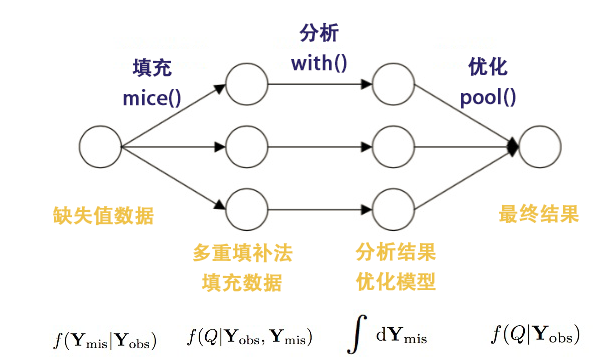1. 填充：mice()函数，从一个包含缺失数据的数据框开始，然后返回一个包含多个完整数据集的对象，每个完整数据集都是通过对原始数据框中的缺失数据进行插而生成的。
2. 分析：with()函数，可依次对每个完整数据集应用统计模型，分析填充的结果。
3. 优化：pool()函数，将这些单独的分析结果整合为一组结果，最终模型的标准误和p值，都将准确地反映出由于缺失值和多重插补而产生的不确定性。

## 4. 填充方法和程序实现

4.1 观察缺失值

``````
> md.pattern(airquality)
Wind Temp Month Day Solar.R Ozone
111    1    1     1   1       1     1  0
35     1    1     1   1       1     0  1
5      1    1     1   1       0     1  1
2      1    1     1   1       0     0  2
0    0     0   0       7    37 44
``````

• 左边第一列，为样本数
• 右边第一列，为累积缺失值个数，0为没有缺失。
• 最下面一行，每个列有多少个缺失值

• rr:行有值，列有值
• rm:行有值，列丢失
• mr:行丢失，列有值
• mm:行丢失，列丢失
``````
> md.pairs(airquality)
\$rr
Ozone Solar.R Wind Temp Month Day
Ozone     116     111  116  116   116 116
Solar.R   111     146  146  146   146 146
Wind      116     146  153  153   153 153
Temp      116     146  153  153   153 153
Month     116     146  153  153   153 153
Day       116     146  153  153   153 153

\$rm
Ozone Solar.R Wind Temp Month Day
Ozone       0       5    0    0     0   0
Solar.R    35       0    0    0     0   0
Wind       37       7    0    0     0   0
Temp       37       7    0    0     0   0
Month      37       7    0    0     0   0
Day        37       7    0    0     0   0

\$mr
Ozone Solar.R Wind Temp Month Day
Ozone       0      35   37   37    37  37
Solar.R     5       0    7    7     7   7
Wind        0       0    0    0     0   0
Temp        0       0    0    0     0   0
Month       0       0    0    0     0   0
Day         0       0    0    0     0   0

\$mm
Ozone Solar.R Wind Temp Month Day
Ozone      37       2    0    0     0   0
Solar.R     2       7    0    0     0   0
Wind        0       0    0    0     0   0
Temp        0       0    0    0     0   0
Month       0       0    0    0     0   0
Day         0       0    0    0     0   0
``````

``````
# 加载VIM包
> library(VIM)
> mice_plot <- aggr(airquality, col=c('navyblue','yellow'),
+                     numbers=TRUE, sortVars=TRUE,
+                     labels=names(airquality), cex.axis=.7,
+                     gap=3, ylab=c("Missing data","Pattern"))

Variables sorted by number of missings:
Variable      Count
Ozone 0.24183007
Solar.R 0.04575163
Wind 0.00000000
Temp 0.00000000
Month 0.00000000
Day 0.00000000

``````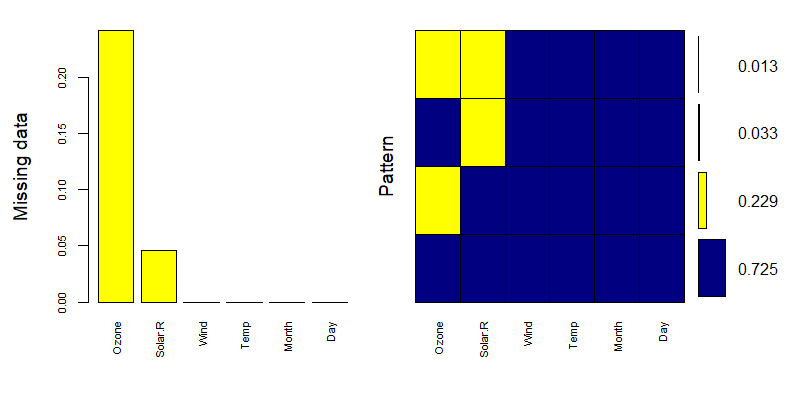``> matrixplot(airquality)``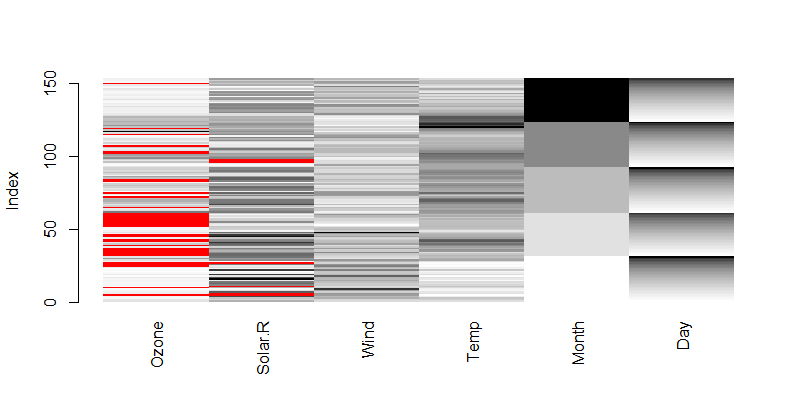``````
> marginplot(airquality[c(1,2)], col = c("blue", "red", "orange"))
``````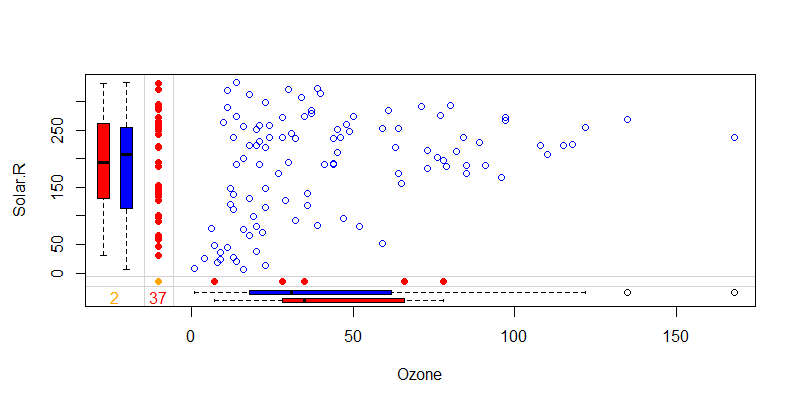x轴为Ozone列，y轴为Solar.R列，蓝色点为数据散点图，红色点分别表示Ozone的缺失值和Solar.R列的缺失值位置。

4.2 多重填补法

``````
# 进行填充
> imputed_Data <- mice(airquality, m=5, maxit = 50, method = 'pmm', seed = 500)
iter imp variable
1   1  Ozone  Solar.R
1   2  Ozone  Solar.R
1   3  Ozone  Solar.R
1   4  Ozone  Solar.R
1   5  Ozone  Solar.R
2   1  Ozone  Solar.R
2   2  Ozone  Solar.R
2   3  Ozone  Solar.R
2   4  Ozone  Solar.R
2   5  Ozone  Solar.R

// 省略输出
``````

• airquality, 原始数据集
• m, 多重填补法的填补矩阵数，默认为5次
• maxit，最大迭代次数，默认5次
• method, 填补用的方法，pmm为预测均值匹配(predictive mean matching)。用methods(mice) 可以看到有哪些可用的方法。

``````
> summary(imputed_Data)
Class: mids
Number of multiple imputations:  5
Imputation methods:
Ozone Solar.R    Wind    Temp   Month     Day
"pmm"   "pmm"      ""      ""      ""      ""
PredictorMatrix:
Ozone Solar.R Wind Temp Month Day
Ozone       0       1    1    1     1   1
Solar.R     1       0    1    1     1   1
Wind        1       1    0    1     1   1
Temp        1       1    1    0     1   1
Month       1       1    1    1     0   1
Day         1       1    1    1     1   0
``````

``````
> imputed_Data\$imp
\$Ozone
1   2   3  4   5
5   18  18  18  6   1
10  20  30  16 16  16
25  18  19   8  6  13
26   4   1  28 19  37
27  14   7  11 18  41
32  59  40  44 23  31
33  36   7  13 65  41
// 省略输出

\$Solar.R
1   2   3   4   5
5  215 299  65  98 112
6  222 157 320 332 279
11 183  47 137 135   7
27  36 223  25 238 238
96 273 323 291 294 175
97 287 275 285  31 237
98 323 187 294 320 264

// 省略输出
``````

``````
> stripplot(imputed_Data, col=c("grey",mdc(2)),pch=c(1,20))``````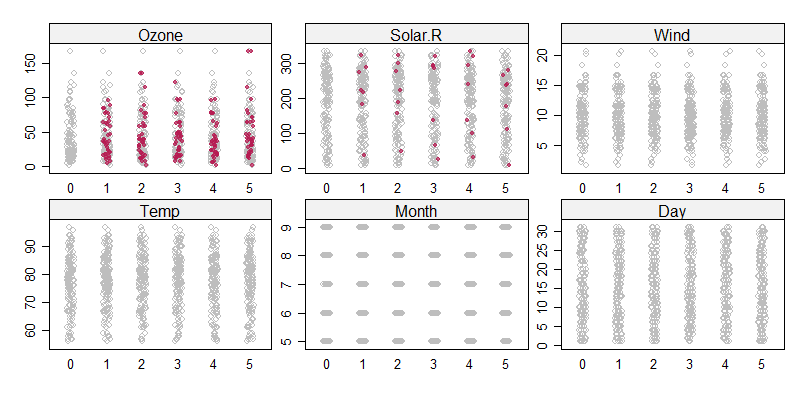``````
> xyplot(imputed_Data , Ozone ~  Solar.R | .imp, pch=20,cex=1.2)
``````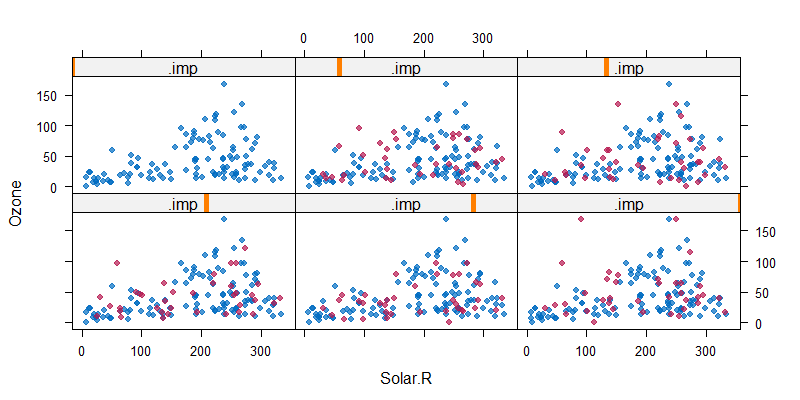3.3 分析结果，优化模型

``````
> fit=with(imputed_Data,lm(Ozone ~ Wind + Solar.R + Temp))

# 查看统计结果
> summary(fit)
# A tibble: 20 x 5
term        estimate std.error statistic  p.value
<chr>          <dbl>     <dbl>     <dbl>    <dbl>
1 (Intercept) -39.9      19.1        -2.09 3.87e- 2
2 Wind         -3.60      0.549      -6.55 8.90e-10
3 Solar.R       0.0572    0.0200      2.87 4.72e- 3
4 Temp          1.38      0.213       6.46 1.39e- 9
5 (Intercept) -69.2      19.7        -3.51 5.99e- 4
6 Wind         -3.24      0.567      -5.71 5.81e- 8
7 Solar.R       0.0582    0.0204      2.86 4.84e- 3
8 Temp          1.71      0.218       7.85 7.50e-13
9 (Intercept) -59.5      19.2        -3.10 2.34e- 3
10 Wind         -3.13      0.550      -5.68 6.85e- 8
11 Solar.R       0.0571    0.0198      2.88 4.54e- 3
12 Temp          1.57      0.215       7.29 1.66e-11
13 (Intercept) -67.4      18.5        -3.64 3.73e- 4
14 Wind         -2.94      0.531      -5.53 1.41e- 7
15 Solar.R       0.0668    0.0189      3.54 5.40e- 4
16 Temp          1.61      0.204       7.87 6.83e-13
17 (Intercept) -38.4      21.6        -1.78 7.69e- 2
18 Wind         -3.99      0.620      -6.44 1.52e- 9
19 Solar.R       0.0523    0.0223      2.34 2.05e- 2
20 Temp          1.44      0.239       6.04 1.15e- 8
``````

• term，变量
• estimate ，参数估计值
• std.error，残差的标准误差
• statistic，t统计量
• p.value，p-value值

4.4 模型评估

``````
> pooled=pool(fit)
> pooled
Class: mipo    m = 5
estimate         ubar            b            t dfcom        df        riv
(Intercept) -54.87825512 3.864370e+02 2.189907e+02 6.492258e+02   149  19.08909 0.68003032
Wind         -3.37959755 3.186764e-01 1.758552e-01 5.297026e-01   149  19.61464 0.66219610
Solar.R       0.05833371 4.121520e-04 2.745861e-05 4.451024e-04   149 114.74908 0.07994702
Temp          1.54135605 4.758977e-02 1.736383e-02 6.842637e-02   149  30.33948 0.43783767
lambda        fmi
(Intercept) 0.40477265 0.45866597
Wind        0.39838627 0.45159195
Solar.R     0.07402865 0.08975652
Temp        0.30451120 0.34623283
``````

• mipo，一种数据结构，pool用于产生mipo对象
• m, m=5 多组插补数据
• estimate，估计值，pool汇总的完整数据估计
• ubar, 估计的内插方差，std.error的平方的均值，越小越好
• b，估计之间的插补方差， estimate的方差，越小越好
• t，估计的总方差，ubar + (1 + 1 / m) * b， 越小越好
• dfcom，完整数据的自由度
• df，t统计量的自由度，由Barnard-Rubin提供一种自由度的计算方法，越小越好
• riv，方差的相对增加，(1 + 1 / m) * b / ubar，越小越好
• lambda, 缺失值的比例，(1 + 1 / m) * b / t，越小越好
• fmi, 缺少信息的部分，(riv + 2 / (df + 3)) / (riv + 1))，越小越好

``````
> pool.r.squared(fit)
est     lo 95     hi 95 fmi
R^2 0.5630547 0.4404848 0.6677567 NaN
``````

• est, 合并的R^2，估计值
• lo 95，合并的R^2，95%下限
• hi 95，合并的R^2，95%上限
• fmi，缺少信息的部分，越小越好

pool的过程，主要就是完成F检验和R^2检验。

3.4 最终结果

``````
> completeData <- complete(imputed_Data,2)
> completeData
Ozone Solar.R Wind Temp Month Day
1      41     190  7.4   67     5   1
2      36     118  8.0   72     5   2
3      12     149 12.6   74     5   3
4      18     313 11.5   62     5   4
5      18     299 14.3   56     5   5
6      28     157 14.9   66     5   6
7      23     299  8.6   65     5   7
8      19      99 13.8   59     5   8
9       8      19 20.1   61     5   9
10     30     194  8.6   69     5  10
``````

mice包提供了29种填充算法，上面的例子我只是用到了pmm一种方法。大家可以根据不同的业务场景要求和数据的分布情况，用不同方法进行填充，让数据差异变化最小。

``````
> methods(mice)
 mice.impute.2l.bin       mice.impute.2l.lmer      mice.impute.2l.norm      mice.impute.2l.pan
 mice.impute.2lonly.mean  mice.impute.2lonly.norm  mice.impute.2lonly.pmm   mice.impute.cart
 mice.impute.jomoImpute   mice.impute.lda          mice.impute.logreg       mice.impute.logreg.boot
 mice.impute.mean         mice.impute.midastouch   mice.impute.norm         mice.impute.norm.boot
 mice.impute.norm.nob     mice.impute.norm.predict mice.impute.panImpute    mice.impute.passive
 mice.impute.rf           mice.impute.ri           mice.impute.sample       mice.mids
 mice.theme
``````

http://blog.fens.me/r-na-mice## 用R语言解读牛顿冷却定律

R的极客理想系列文章，涵盖了R的思想，使用，工具，创新等的一系列要点，以我个人的学习和体验去诠释R的强大。

R语言作为统计学一门语言，一直在小众领域闪耀着光芒。直到大数据的爆发，R语言变成了一门炙手可热的数据分析的利器。随着越来越多的工程背景的人的加入，R语言的社区在迅速扩大成长。现在已不仅仅是统计领域，教育，银行，电商，互联网….都在使用R语言。

• 张丹(Conan), 程序员/Quant: Java,R,Nodejs
• blog: http://blog.fens.me
• email: bsspirit@gmail.com1. 牛顿冷却定律
2. 数据集
3. 程序实现

## 1. 牛顿冷却定律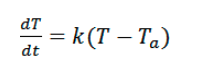• T: 温度
• t: 时间
• T(t)：t时刻的温度
• Ta:代表室温，T(t)-Ta就是当前温度与室温之间的温差。当前温度高于室温时，T(t)-Ta > 0。
• T0:初始温度
• k:传热系数，常数，表示室温与降温速率之间的比例关系
• dT/dt:温度和时间的微分形式，表示物体的温度随时间下降的速度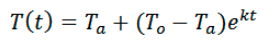## 2. 数据集

• Win10 64bit
• R: 3.6.1 x86_64-w64-mingw32/x64 b4bit

• time_min：分钟，冷却时间，从0分钟到60分钟
• temp_100ml：温度(摄氏度)，100ml水随时间变化的温度
• temp_300ml：温度(摄氏度)，300ml水随时间变化的温度
• temp_800ml：温度(摄氏度)，800ml水随时间变化的温度
``````
> dat
time_min temp_100ml temp_300ml temp_800ml
1         0        100        100        100
2         1         95         95         96
3         2         82         91         95
4         3         79         87         92
5         4         74         84         90
6         5         70         81         88
7         6         67         78         85
8         7         65         76         83
9         8         61         73         80
10        9         59         71         78
11       10         57         70         76
12       11         56         68         75
13       12         54         66         74
14       13         52         64         73
15       14         51         63         71
16       15         50         61         70
17       16         49         60         68
18       17         48         58         66
19       18         47         58         66
20       19         45         56         65
21       20         45         55         63
22       21         44         55         62
23       22         43         54         61
24       23         42         53         60
25       24         42         52         60
26       25         41         51         59
27       26         41         50         58
28       27         40         49         56
29       28         39         48         56
30       29         38         48         55
31       30         38         47         54
32       31         38         46         53
33       32         38         46         52
34       33         37         45         52
35       34         36         45         51
36       35         36         45         50
37       40         34         42         47
38       45         33         40         45
39       50         31         38         43
40       55         30         37         41
41       60         29         36         40
``````

``````
# 加载数据集

# 以散点图观察数据
> plot(dat\$time_min,dat\$temp_100ml)
> points(dat\$time_min,dat\$temp_300ml,col="red")
> points(dat\$time_min,dat\$temp_800ml,col="blue")
``````
• x轴为时间
• y轴为维度
• 黑色散点，100毫升水的温度变化
• 红色散点，300毫升水的温度变化
• 蓝色散点，800毫升水的温度变化

## 3. 程序实现

3.1 用牛顿冷却定律计算100毫升水的温度

1. 计算1分钟内的k值
2. 计算每个统计时间的k值，并求平均k值
3. 根据平均k值，计算每个统计时间温度
4. 对比理论值和测量值，进行可视化
5. 计算理论值和测量值的均方根误差
6. 进行理论值和测量值的统计检验
7. 得出结论

``````
# 室温 23度
> Ta <- 23

# 根据公式计算k值, k = ln((T(1)-Ta)/(T(0)-Ta))/t1
> k = log((dat\$temp_100ml-Ta)/(dat\$temp_100ml-Ta)) / dat\$time_min
> k
 -0.0671393
``````

``````
# 每个统计时候的k值
> kv = log((dat\$temp_100ml[-1]-Ta)/(dat\$temp_100ml-Ta)) / dat\$time_min[-1]
> kv
 -0.06713930 -0.13313399 -0.10615124 -0.10299495 -0.09873156 -0.09326930 -0.08659083
 -0.08827741 -0.08447628 -0.08174449 -0.07702708 -0.07581818 -0.07511612 -0.07225721
 -0.06986457 -0.06785681 -0.06617233 -0.06476398 -0.06593489 -0.06263815 -0.06187062
 -0.06127605 -0.06084202 -0.05830694 -0.05813735 -0.05590129 -0.05594785 -0.05611488
 -0.05640535 -0.05452517 -0.05276630 -0.05111735 -0.05165903 -0.05231930 -0.05082446
 -0.04864775 -0.04536045 -0.04528728 -0.04359810 -0.04253410

# k平均值
> k<-mean(kv);k
 -0.06758501
``````

``````
# 计算理论温度
> Tt<- Ta + (dat\$temp_100ml - Ta)*exp(k*dat\$time_min[-1])
> Tt
 94.96792 90.26469 85.86882 81.76024 77.92015 74.33103 70.97645 67.84111 64.91067 62.17173
 59.61179 57.21915 54.98287 52.89273 50.93919 49.11331 47.40676 45.81174 44.32095 42.92759
 41.62529 40.40809 39.27044 38.20714 37.21333 36.28446 35.41630 34.60487 33.84648 33.13764
 32.47513 31.85591 31.27716 30.73624 30.23066 28.15726 26.67841 25.62362 24.87129 24.33470
``````

``````
# 把100ml的理论值和测量值合并数据框
> df100<-data.frame(dat[,1:2],Tt=c(100,Tt))

# 进行可视化，测试值为黑色点，理论值为红色线
> plot(df100\$time_min,df100\$temp_100ml,ylim=c(0,100))
> lines(df100\$time_min,df100\$Tt,col="red")
``````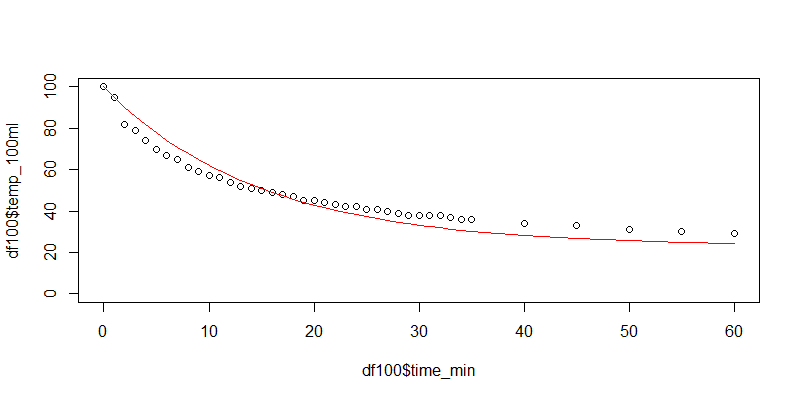``````
> sqrt(sum((df100\$Tt - df100\$temp_100ml)^2)/nrow(df100))
 4.800323
``````

``````
# 观测值检验
> shapiro.test(df100[,2])

Shapiro-Wilk normality test

data:  df100[, 2]
W = 0.88026, p-value = 0.0004557

# 理论值检验
> shapiro.test(df100[,3])

Shapiro-Wilk normality test

data:  df100[, 3]
W = 0.90219, p-value = 0.001925
``````

``````
# 卡方检验
> x <- matrix(c(df100[,2],df100[,3]), ncol = 2)
> chisq.test(x)

Pearson's Chi-squared test

data:  x
X-squared = 10.266, df = 40, p-value = 1
``````

3.2 用牛顿冷却定律计算300毫升水的温度
300毫升水，计算理论值。

``````
# 300毫升水
> kv = log((dat\$temp_300ml[-1]-Ta)/(dat\$temp_300ml-Ta)) / dat\$time_min[-1]

# k均值
> k<-mean(kv);k
 -0.0447057

# 计算每个统计时间的温度
> Tt<- Ta + (dat\$temp_300ml - Ta)*exp(k*dat\$time_min[-1]);Tt
 96.63347 93.41413 90.33555 87.39156 84.57629 81.88411 79.30963 76.84771 74.49342 72.24207
 70.08915 68.03036 66.06159 64.17888 62.37850 60.65682 59.01043 57.43601 55.93043 54.49067
 53.11386 51.79725 50.53820 49.33420 48.18284 47.08182 46.02894 45.02208 44.05925 43.13852
 42.25804 41.41606 40.61089 39.84092 39.10461 35.87873 33.29902 31.23605 29.58630 28.26701

# 合并理论值和观测值
> df300<-data.frame(dat[,c('time_min',"temp_300ml")],Tt=c(100,Tt))

# 均方根误差
> sqrt(sum((df300\$Tt - df300\$temp_300ml)^2)/nrow(df300))
 3.711398

# 可视化
> plot(df300\$time_min,df300\$temp_300ml,ylim=c(0,100))
> lines(df300\$time_min,df300\$Tt,col="red")
``````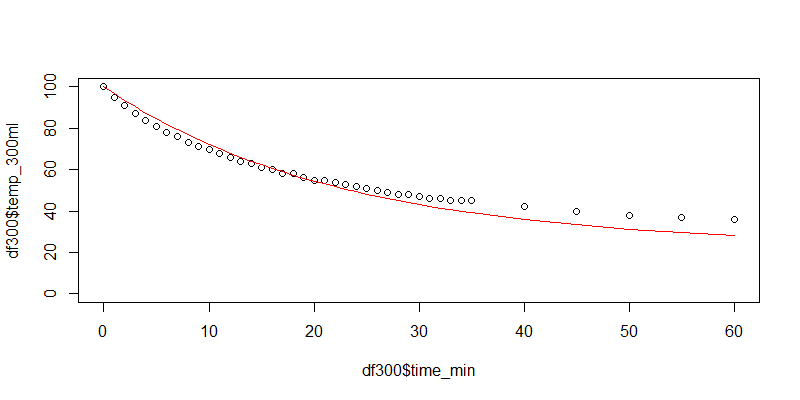3.3 用牛顿冷却定律计算800毫升水的温度
800毫升水，计算理论值

``````
> kv = log((dat\$temp_800ml[-1]-Ta)/(dat\$temp_800ml-Ta)) / dat\$time_min[-1]

# k均值
> k<-mean(kv);k
 -0.03266545

# 计算每个统计时间的温度
> Tt<- Ta + (dat\$temp_800ml - Ta)*exp(k*dat\$time_min[-1]);Tt
 97.52540 95.13032 92.81222 90.56862 88.39712 86.29540 84.26123 82.29244 80.38692 78.54263
 76.75762 75.02998 73.35785 71.73947 70.17309 68.65706 67.18974 65.76959 64.39507 63.06473
 61.77714 60.53093 59.32478 58.15738 57.02750 55.93394 54.87552 53.85111 52.85963 51.90001
 50.97123 50.07230 49.20226 48.36018 47.54516 43.84653 40.70523 38.03729 35.77137 33.84689

# 合并理论值和观测值
> df800<-data.frame(dat[,c('time_min',"temp_800ml")],Tt=c(100,Tt))

# 均方根误差
> sqrt(sum((df800\$Tt - df800\$temp_800ml)^2)/nrow(df800))
 2.237821

# 可视化
plot(df800\$time_min,df800\$temp_800ml,ylim=c(0,100))
lines(df800\$time_min,df800\$Tt,col="red")
``````

3.4 总结

 水量 k平均值 均方根误差 100ml -0.06758501 4.800323 300ml -0.0447057 3.711398 800ml -0.03266545 2.237821

800ml的烧瓶的水，均方根误差最小，理论值与观测值拟合最好；同时，传热系数k值最小，说明水量越多传热越慢。100ml的烧杯的水，均方根误差最大，理论值与观测值拟合最差；同时，传热系数k值最大，说明水量越少传热越快。## 用R语言解读统计检验-卡方检验

R的极客理想系列文章，涵盖了R的思想，使用，工具，创新等的一系列要点，以我个人的学习和体验去诠释R的强大。

R语言作为统计学一门语言，一直在小众领域闪耀着光芒。直到大数据的爆发，R语言变成了一门炙手可热的数据分析的利器。随着越来越多的工程背景的人的加入，R语言的社区在迅速扩大成长。现在已不仅仅是统计领域，教育，银行，电商，互联网….都在使用R语言。

• 张丹(Conan), 程序员/Quant: Java,R,Nodejs
• blog: http://blog.fens.me
• email: bsspirit@gmail.com

http://blog.fens.me/r-test-x2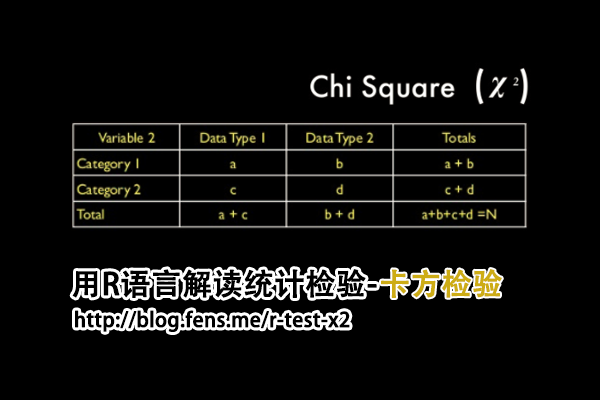1. 卡方检验介绍
2. 数据集
3. 四格表法推导过程
4. 程序实现

## 1. 卡方检验介绍

• χ2，为统计量，用于衡量实际值与理论值的差异程度。
• A，为实际值
• T，为理论值
• 自由度，df = n-1

## 2. 数据集

• Win10 64bit
• R: 3.6.1 x86_64-w64-mingw32/x64 b4bit

``````
> N=100                 # 抛硬币100次
> set.seed(1)           # 随机种子
> coin<-sample(x=c(0,1), prob=c(0.5,0.5),size = N, replace = TRUE)  # 模拟抛硬币
> coin                  # 打印结果
 1 1 0 0 1 0 0 0 0 1 1 1 0 1 0 1 0 0 1 0 0 1 0 1 1 1 1 1 0 1 1 0 1 1 0 0 0 1 0 1 0 0 0 0
 0 0 1 1 0 0 1 0 1 1 1 1 1 0 0 1 0 1 1 1 0 1 1 0 1 0 1 0 1 1 1 0 0 1 0 0 1 0 1 1 0 1 0 1
 1 1 1 1 0 0 0 0 1 1 0 0
``````

``````
> table(coin)  # 统计0和1的出现次数
coin
0  1
48 52
``````

``````
> cnt <- cumsum(coin)  # 积累求和，正面1出现的次数
> n<-1:N               # 次数向量
> rate<- cnt/1:N       # 累积的正面出现概率
> plot(n,rate,type="o",log="x",   # 画图，x轴做log变型
+      xlim=c(1,N), ylim=c(0.0,1.0), cex.axis=1.5,
``````

## 3. 四格表法推导过程

 正面 反面 合计 观察次数 52 48 100 期望次数 50 50 100 合计 102 98 200

 正面 反面 合计 观察次数 100*0.51 =51 100*(1-0.51) = 49 100 期望次数 100*0.51 =51 100*(1-0.51) = 49 100 合计 102 98 200

``````
X^2 = (52- 51)^2 / 51 + (48 - 49)^2 / 49 + (50 - 51)^2 / 51 + (50 - 49)^2 / 49 = 0.08003201
``````

1. 所有的理论数T≥5并且总样本量n≥40，用Pearson卡方进行检验.
2. 如果理论数T＜5但T≥1,并且n≥40，用连续性校正的卡方进行检验.
3. 如果有理论数T＜1或n＜40，则用Fisher’s检验.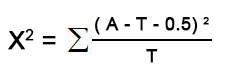``````
X^2 = (abs(52- 51)-0.5)^2 / 51 + (abs(48 - 49)-0.5)^2 / 49 + (abs(50 - 51)-0.5)^2 / 51 + (abs(50 - 49)-0.5)^2 / 49
= 0.020008
``````

## 4. 程序实现

``````
# 合并观测值和期望值，形成矩阵
> s<-c(table(coin),c(50,50))
> x <- matrix(s, ncol = 2, byrow=TRUE)
> x
[,1] [,2]
[1,]   48   52
[2,]   50   50

# 卡方检验
> chisq.test(x)

Pearson's Chi-squared test with Yates' continuity correction

data:  x
X-squared = 0.020008, df = 1, p-value = 0.8875
``````

• H0:抛硬币出现正面和反面的概率都是50%，与次数无关
• X-squared统计量：0.020008
• df，自由度，1
• p-value值：0.8875

``````
# 查看观察值
> chi\$observed
[,1] [,2]
[1,]   48   52
[2,]   50   50

# 查看期望值
> chi\$expected
[,1] [,2]
[1,]   49   51
[2,]   49   51

# 残差
> chi\$residuals
[,1]      [,2]
[1,] -0.1428571  0.140028
[2,]  0.1428571 -0.140028
``````

``````
# 手动计算X^2值
> sr <- rowSums(x)
> sc <- colSums(x)
> n <- sum(x)
> E <- outer(sr, sc, "*")/n;E
[,1] [,2]
[1,]   49   51
[2,]   49   51
> x2 <- sum((abs(x - E) - 0.5)^2/E);x2
 0.020008

# 手动计算P值
> pchisq(x2, 1, lower.tail = FALSE)
 0.8875147
``````

http://blog.fens.me/r-test-x2## 用R语言解读统计检验-F检验

R的极客理想系列文章，涵盖了R的思想，使用，工具，创新等的一系列要点，以我个人的学习和体验去诠释R的强大。

R语言作为统计学一门语言，一直在小众领域闪耀着光芒。直到大数据的爆发，R语言变成了一门炙手可热的数据分析的利器。随着越来越多的工程背景的人的加入，R语言的社区在迅速扩大成长。现在已不仅仅是统计领域，教育，银行，电商，互联网….都在使用R语言。

• 张丹(Conan), 程序员/Quant: Java,R,Nodejs
• blog: http://blog.fens.me
• email: bsspirit@gmail.com

http://blog.fens.me/r-test-f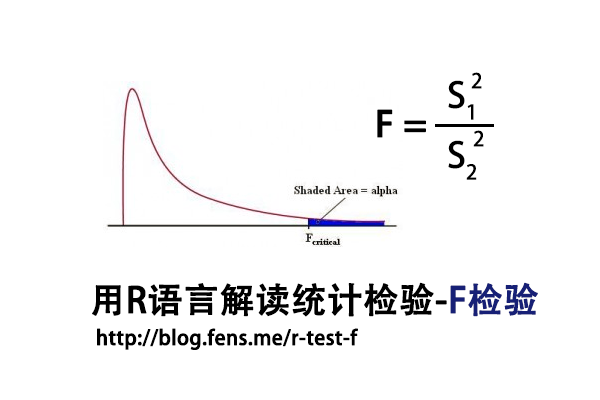1. F检验介绍
2. 数据集
3. F检验实现

## 1. F检验介绍

F检验法(F-test)，初期叫方差比率检验(Variance Ratio)，又叫联合假设检验(Joint Hypotheses Test)，是英国统计学家Fisher提出的，主要通过比较两组数据的方差，以确定他们的密度是否有显著性差异。至于两组数据之间是否存在系统误差，则在进行F检验并确定它们的密度没有显著性差异之后，再进行T检验

F检验，是一种在零假设(H0)之下，统计值服从F-分布的检验。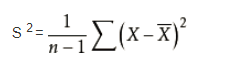F统计量计算公式：

• F:统计量，根据自由度查表，当F值小于查表值时没有显著差异，当F值大于等于查表值时有显著差异
• S1:样本1的标准差
• S2:样本2的标准差
• 分子自由度: df=分子的数量-1
• 分母自由度: df=分母的数量-1

T检验和F检验对比
T检验用来检测数据的准确度(系统误差)，F检验用来检测数据的精密度(偶然误差)。在定量分析过程中，常遇到两种情况：一种是样本测量的平均值与真值不一致；另一种是两组测量的平均值不一致。

T检验是检查两组均值的差异，而F检验是检查多组均值之间的差异。

## 2. 数据集

F检验，对于数据有比较严格的要求，所以我们需要先找到一个合适的数据集，作为测试数据集。我发现了R语言自带的一个数据集ToothGrowth，是很好的测试数据集，本文接下来的内容，将以这个数据集进行测试，来介绍F检验。

• Win10 64bit
• R: 3.4.2 x86_64-w64-mingw32/x64 b4bit

• len列，为牙齿长度
• supp列，为注射方法
• dose列，为注射剂量

``````
len supp dose
1   4.2   VC  0.5
2  11.5   VC  0.5
3   7.3   VC  0.5
4   5.8   VC  0.5
5   6.4   VC  0.5
6  10.0   VC  0.5
7  11.2   VC  0.5
8  11.2   VC  0.5
9   5.2   VC  0.5
10  7.0   VC  0.5
``````

F检验对于数据的正态性非常敏感，我们需要先对选定数据集进行进行正态分布检验。使用Shapiro-Will作为正态分布检验的方法，原假设H0:样本符合正态分布。

``````
# 按不同的处理方法，进行分组
> len_VC<-ToothGrowth\$len[which(ToothGrowth\$supp=='VC')]
> len_OJ<-ToothGrowth\$len[which(ToothGrowth\$supp=='OJ')]

# 正态分布检验
> shapiro.test(len_VC)

Shapiro-Wilk normality test

data:  len_VC
W = 0.96567, p-value = 0.4284

# 正态分布检验
> shapiro.test(len_OJ)

Shapiro-Wilk normality test

data:  len_OJ
W = 0.91784, p-value = 0.02359
``````

``````
> coplot(len ~ dose | supp, data = ToothGrowth, panel = panel.smooth,
xlab = "ToothGrowth data: length vs dose, given type of supplement")
``````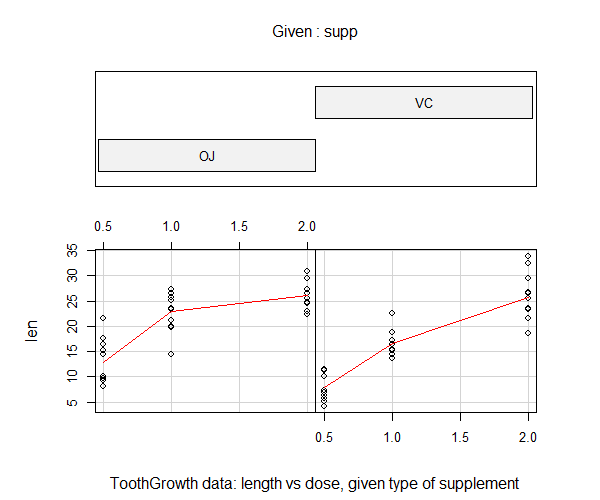## 3. F检验实现

3.1 随机数进行F检验

``````
# 生成随机数
> set.seed(1)
> x <- rnorm(50, mean = 0, sd = 2)
> y <- rnorm(30, mean = 1, sd = 1)

# 进行F检验
> var.test(x, y)

F test to compare two variances

data:  x and y
F = 2.6522, num df = 49, denom df = 29, p-value
= 0.006232
alternative hypothesis: true ratio of variances is not equal to 1
95 percent confidence interval:
1.332510 4.989832
sample estimates:
ratio of variances
2.652168
``````

• H0:原假设2组样本的方差，无显著差异
• F统计量：2.6522
• num df，分子自由度，50-1=49
• denom df，分每自由度，30-1=29
• p-value值：0.006232
• 95 percent confidence interval：95%的置信区间
• ratio of variances：方差比率2.652168

3.2 ToothGrowth进行F检验

``````
> var.test(len_VC,len_OJ)

F test to compare two variances

data:  len_VC and len_OJ
F = 1.5659, num df = 29, denom df = 29, p-value
= 0.2331
alternative hypothesis: true ratio of variances is not equal to 1
95 percent confidence interval:
0.745331 3.290028
sample estimates:
ratio of variances
1.565937
``````

``````
# 手动计算T值
> Xn<-length(len_VC)
> Yn<-length(len_OJ)
> Xm<-mean(len_VC)
> Ym<-mean(len_OJ)

# 计算两组样本的偏方差
> fx<-sum((len_VC-Xm)^2)/(Xn-1)
> fy<-sum((len_OJ-Ym)^2)/(Yn-1)

# 计算F值
> fx/fy
 1.565937

# 手动计算P值，双边检验
> p_value<-pf(f_stat,Yn-1,Xn-1)
> p_value<-2*min(p_value, 1 - p_value);p_value
 0.2331433
``````

http://blog.fens.me/r-test-f## 用R语言解读统计检验-T检验

R的极客理想系列文章，涵盖了R的思想，使用，工具，创新等的一系列要点，以我个人的学习和体验去诠释R的强大。

R语言作为统计学一门语言，一直在小众领域闪耀着光芒。直到大数据的爆发，R语言变成了一门炙手可热的数据分析的利器。随着越来越多的工程背景的人的加入，R语言的社区在迅速扩大成长。现在已不仅仅是统计领域，教育，银行，电商，互联网….都在使用R语言。

• 张丹(Conan), 程序员/Quant: Java,R,Nodejs
• blog: http://blog.fens.me
• email: bsspirit@gmail.com

http://blog.fens.me/r-test-t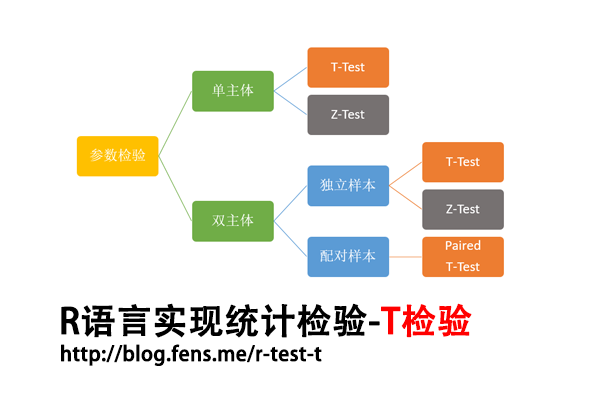1. T检验介绍
2. 数据集
3. 单总体T检验
4. 双总体T检验

## 1. T检验介绍

T检验，也称 student t检验（Student’s t test），是戈斯特为了观测酿酒质量发明的。戈斯特于1908年在Biometrika上公布T检验，但因其老板认为其为商业机密而被迫使用笔名（学生）。

T检验，是用于检验两个小样本的平均值差异程度的检验方法，样本量在3-30个左右，要求样本为正态分布，但总体标准差可未知。T检验，是用T分布理论来推断差异发生的概率，从而判定两个样本平均值的差异是否显著。T检验可分为单总体T检验，双总体非配对T检验，和双总体配对T检验。下面将分别进行介绍。

## 2. 数据集

T检验，对于数据有比较严格的要求，所以我们需要先找到一个合适的数据集，作为测试数据集。我发现了R语言自带的一个数据集sleep，是很好的测试数据集，本文接下来的内容，将以这个数据集进行测试，来介绍T检验。

• Win10 64bit
• R: 3.4.2 x86_64-w64-mingw32/x64 b4bit

• extra列，为睡眠时间的变化
• group列，为药物编号
• ID列，为患者编号

``````
> data(sleep)
> sleep
extra group ID
1    0.7     1  1
2   -1.6     1  2
3   -0.2     1  3
4   -1.2     1  4
5   -0.1     1  5
6    3.4     1  6
7    3.7     1  7
8    0.8     1  8
9    0.0     1  9
10   2.0     1 10
11   1.9     2  1
12   0.8     2  2
13   1.1     2  3
14   0.1     2  4
15  -0.1     2  5
16   4.4     2  6
17   5.5     2  7
18   1.6     2  8
19   4.6     2  9
20   3.4     2 10
``````

Shapiro-Wilk正态分布检验: 用来检验是否数据符合正态分布，类似于线性回归的方法一样，是检验其于回归曲线的残差。该方法推荐在样本量很小的时候使用，样本在3到5000之间。该检验原假设为H0:数据集符合正态分布，统计量为W。统计量W最大值是1，越接近1，表示样本与正态分布匹配。p值，当p-value小于显著性水平α(0.05)，则拒绝H0。

``````
> shapiro.test(sleep\$extra) # 总体正态分布

Shapiro-Wilk normality test

data:  sleep\$extra
W = 0.94607, p-value = 0.3114
``````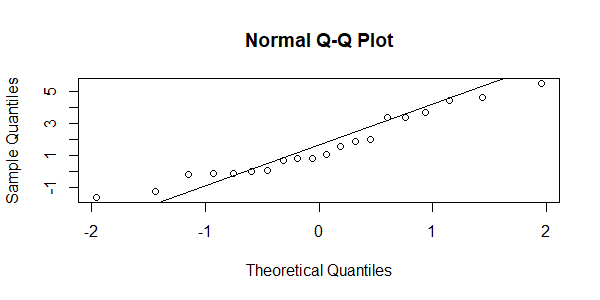## 3. 单总体的T检验

1. 已知总体均值Um
2. 可得到样本均值Xm，及该样本标准误差Xs
3. 样本来自正态或近似正态总体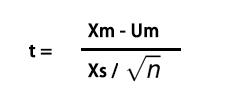• Xm：样本均值
• Um：总体均值
• Xs：样本标准差
• n：样本个数
• 自由度：df=n-1

``````
#  总体均值
> Um<-mean(sleep\$extra)

# 取group=1的样本组
> g1<-sleep\$extra[which(sleep\$group==1)]

# 计算样本均值，方差，数量
> Xm<-mean(g1)
> Xs<-sd(g1)
> n<-length(g1)

# 进行T检验
> t.test(g1,mu=Um)

One Sample t-test

data:  g1
t = -1.3964, df = 9, p-value = 0.1961
alternative hypothesis: true mean is not equal to 1.54
95 percent confidence interval:
-0.5297804  2.0297804
sample estimates:
mean of x
0.75

``````

• H0:原假设总体和样本均值无显著差异
• t统计量：-1.3964
• df，自由度，10-1=9
• p-value值：0.1961
• 95 percent confidence interval：95%的置信区间
• mean：样本均值0.75

``````
# 手动计算T值
> t_stat<-(Xm-Um) / (Xs/(sqrt(n)));t_stat
 -1.396415

# 手动计算P值
> p_value<-2*pt(-abs(t_stat),df=n-1);p_value
 0.1960699
``````

## 4. 双总体T检验

4.1 配对T检验

• 两种同质样本，分别接受两种不同的处理，如性别、年龄、体重、病情程度相同进行配对。
• 同一样本或同一样本的两个部分，分别接受两种不同的处理。
• 同一样本自身对比，把同一组样本处理前后的结果进行比较。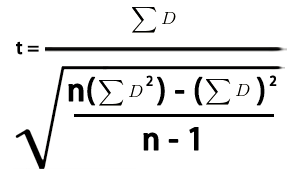• D：两个样本差值
• εD：求和
• ε(D^2)：平方和
• n：样本个数
• 自由度：df=n-1

``````
# 配对T检验
> t.test(extra ~ group, data = sleep, paired = TRUE)

Paired t-test

data:  extra by group
t = -4.0621, df = 9, p-value = 0.002833
alternative hypothesis: true difference in means is not equal to 0
95 percent confidence interval:
-2.4598858 -0.7001142
sample estimates:
mean of the differences
-1.58
``````

``````
# 生成数据集
> g1<-sleep\$extra[which(sleep\$group==1)]
> g2<-sleep\$extra[which(sleep\$group==2)]

# 配对T检验，与上面的计算结果是一致的
> t.test(g1,g2,paired=TRUE)

Paired t-test

data:  g1 and g2
t = -4.0621, df = 9, p-value = 0.002833
alternative hypothesis: true difference in means is not equal to 0
95 percent confidence interval:
-2.4598858 -0.7001142
sample estimates:
mean of the differences
-1.58

# 动手计算
> n1<-length(g1)
> n2<-length(g2)
> D<-g1-g2
> Ds<-sum(D)

# 计算T值
> Ds/sqrt((n1*sum(D^2)-sum(D)^2)/(n1-1))
 -4.062128
``````

4.2 非配对T检验

• n1 和n2 为两样本容量
• Xm1和Xm2为两样本均值
• Xs1和Xs2为两样本的标准差

``````
> t.test(extra ~ group, data = sleep)

Welch Two Sample t-test

data:  extra by group
t = -1.8608, df = 17.776, p-value = 0.07939
alternative hypothesis: true difference in means is not equal to 0
95 percent confidence interval:
-3.3654832  0.2054832
sample estimates:
mean in group 1 mean in group 2
0.75            2.33
``````

``````
# 计算数量，均值，标准差
> n1<-length(g1)
> n2<-length(g2)
> Xm1<-mean(g1)
> Xm2<-mean(g2)
> Xs1<-sd(g1)
> Xs2<-sd(g2)

# 计算T值
> (Xm1-Xm2)/sqrt((Xs1^2/n1)+(Xs2^2/n2))
 -1.860813
``````

``````
> boxplot(extra~group,data=sleep)
``````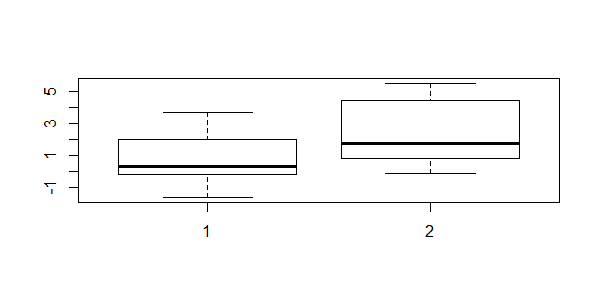http://blog.fens.me/r-test-t